•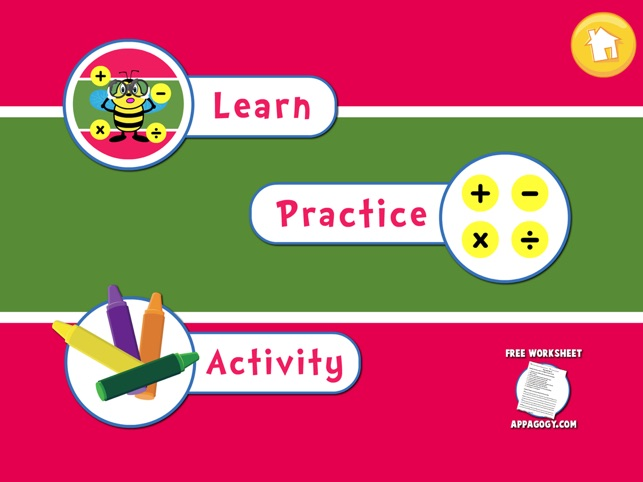•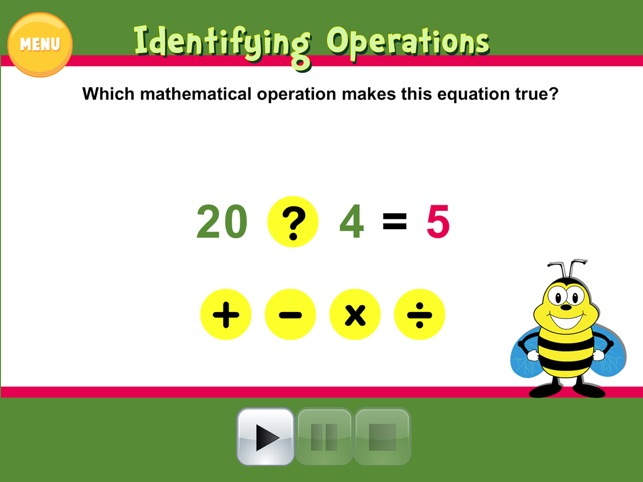•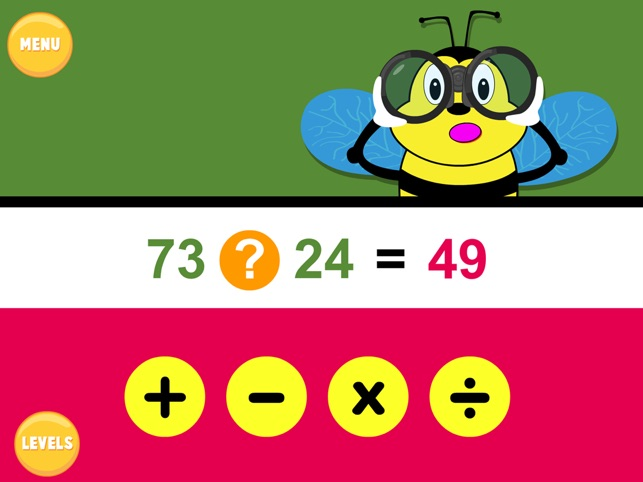•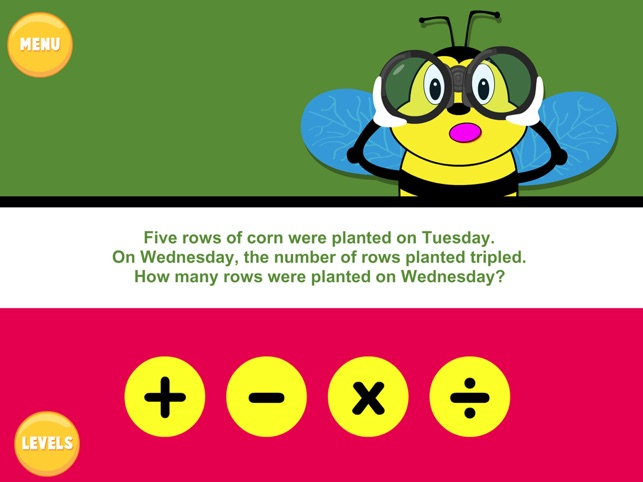•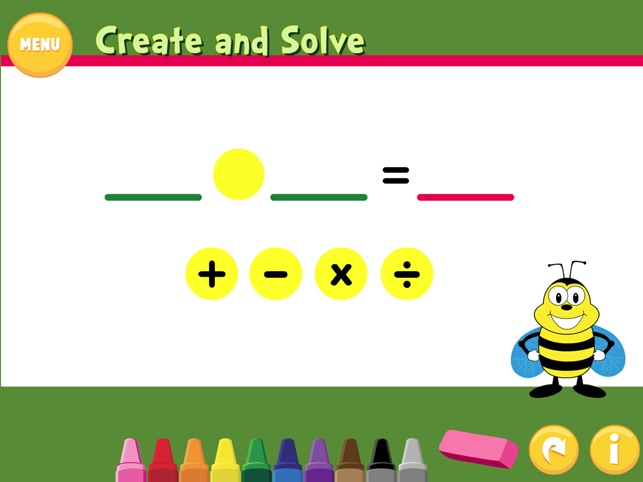## Description

Learn how to identify math operations with this teacher/parent guided, standards aligned lesson.

Students will:
• Identify four types of operations, their symbols and when to use them
• Practice addition, subtraction, multiplication and division math facts
• Read word problems and determine which operation to use to solve
• Create and solve problems for four types of operations

3 4 5

Common Core Standards
3.NBT.3 Multiply one-digit whole numbers by multiples of 10 in the range 10‚ 90 (e.g., 9 * 80, 5 * 60) using strategies based on place value and properties of operations.

3.OA.1 Interpret products of whole numbers, e.g., interpret 5 * 7 as the total number of objects in 5 groups of 7 objects each. For example, describe a context in which a total number of objects can be expressed as 5 * 7.

3.OA.2 Interpret whole-number quotients of whole numbers, e.g., interpret 56 * 8 as the number of objects in each share when 56 objects are partitioned equally into 8 shares, or as a number of shares when 56 objects are partitioned into equal shares of 8 objects each. For example, describe a context in which a number of shares or a number of groups can be expressed as 56 * 8.

3.OA.3 Use multiplication and division within 100 to solve word problems in situations involving equal groups, arrays, and measurement quantities, e.g., by using drawings and equations with a symbol for the unknown number to represent the problem.

3.OA.4 Determine the unknown whole number in a multiplication or division equation relating three whole numbers. For example, determine the unknown number that makes the equation true in each of the equations 8 ? = 48, 5 = * * 3, 6 * 6 = ?.

3.OA.5 Apply properties of operations as strategies to multiply and divide.2 Examples: If 6 * 4 = 24 is known, then 4 * 6 = 24 is also known. (Commutative property of multiplication.) 3 * 5 * 2 can be found by 3 5 = 15, then 15 * 2 = 30, or by 5 * 2 = 10, then 3 * 10 = 30. (Associative property of multiplication.) Knowing that 8 * 5 = 40 and 8 * 2 = 16, one can find 8 * 7 as 8 * (5 + 2) = (8 * 5) + (8 * 2) = 40 + 16 = 56. (Distributive property.)

## What’s New

Version 2.0

• Word problem level added
• User interface upgraded for easy navigation
• IOS 9 compatible
• Group or partner activity for the classroom

## Information

Seller
Kerrie GALLAGHER
Size
20.2 MB
Category
Education
Compatibility

Requires iOS 5.1.1 or later. Compatible with iPad.

Languages

English, Simplified Chinese, Traditional Chinese

Age Rating
Rated 4+
•